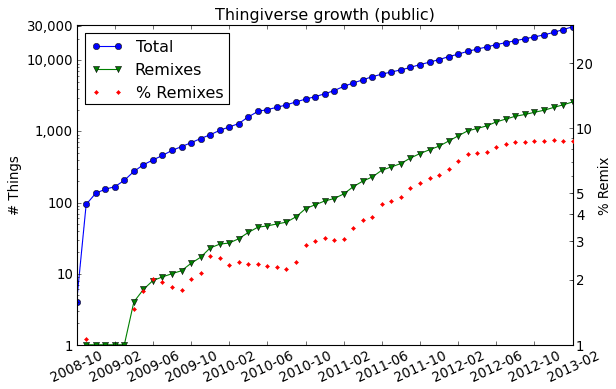## Towards laws of the 3D printable design web

With the explosive growth of 3D printing, and rapid manufacturing at the consumer level in general, physical objects can be designed and manipulated in a computer. However, like other forms of digital content (e.g., documents, software, music), this is only part of the story: digital representation also enables online sharing and collaboration (as Chris Anderson has pointed out). A prime example of the potential of all these technologies combined with online sharing and collaboration is the initial design of consumer-grade 3D printers themselves which, perhaps unsurprisingly, was what many early adopters of the technology used it for.  Considering that the rest of us is where those early adopters were five or more years ago, the future should be interesting.

Despite hearing about 3D printing daily, very few studies have looked at the digital content of physical things, and the processes that generate it. I collected data some time ago, and started off with this visualization, which I wrote about before. A further initial analysis of the data has some interesting stories to tell.

Exponential growth rates.  The total number of things over time (blue) exhibits an exponential growing, with a compound doubling time of 6.1 months. Furthermore, if we consider only remixes (green), then the growth rate far outpaces the overall rate, with a compound doubling time of 4.6 months. Consequently, the relative ratio of remixes is also growing at an exponential pace (red) and, although obviously this cannot continue forever, there is little evidence that the growth rate of remixing is abating (in fact, after the introduction of the Thingiverse Customizer, which is excluded from this plot, the rate has picked up even further).Popularity: views vs. likes vs. makes.  The following table summarizes the results of least-squares regression on measures of user actions, showing the top-3 best predictive features ($$p < 0.01$$, ranked by $$t$$-test scores) with 95% confidence intervals of the corresponding regression coefficients, as well as the bottom-2 worst features.

Variable Best predictors Worst predictors
$$\mathit{\#Views}$$ $$\mathit{\#Likes}\!: 43.1\text{–}44.6, \mathit{\#DLs}\!: 0.35\text{–}0.38, \mathit{\#Views}’\!: 0.28\text{–}0.31$$ $$\mathit{\#Make}’\, (p=0.48), \mathit{\#Remix}’\, (p=0.06)$$
$$\mathit{\#DLs}$$ $$\mathit{\#Likes}\!: 43.1\text{–}44.6, \mathit{\#DLs}\!: 0.35\text{–}0.38, \mathit{\#Views}’\!: 0.28\text{–}0.31$$ $$\mathit{\#Remix}\, (p=0.66), \mathit{\#Remix}’\, (p=0.51)$$
$$\mathit{\#Likes}$$ $$\mathit{\#Views}\!: 0.006, \mathit{\#Make}\!: 2.72\text{–}2.83, \mathit{\#Likes}’\!: 0.42\text{–}0.46$$ $$\mathit{\#Remix}’\, (p=0.59), \mathit{\#DLs}’\, (p=0.27)$$
$$\mathit{\#Makes}$$ $$\mathit{\#Likes}\!: 0.074\text{–}0.077, \mathit{\bf\#Files}\!: -0.13\text{–}0.11, \mathit{\#Makes}’\!: 0.28\text{–}0.33$$ $$\mathit{\#Remix}’\, (p=0.99), \mathit{\#DLs}’\, (p=0.51)$$
$$\mathit{\#Remix}$$ $$\mathit{\#Views}\!: 0.0003, \mathit{\bf\#Remix}’\!: 0.18\text{–}0.27, \mathit{\bf\#Sources}\!: 0.19\text{–}0.39$$ $$\mathit{\bf\#Make}’\, (p=0.71), \mathit{\#DLs}\, (p=0.66)$$

The relative incidence of user actions depends on the relative effort required to take those actions. Therefore, we observe that roughly (order of magnitude) 100 views “contribute” one like in our linear models, and roughly 10 likes “contribute” a make. The first is not particularly surprising. However, the fact that only 10$$\times$$ likes contribute a make seems to suggest that users are actively seeking things, and have the means and motivation to actually print things that they have liked.

Another intuitive, in retrospect, observation is that the number of files has a negative effect on makes. This provides evidence for the hypothesis that simpler things (consisting of fewer parts) are more likely to be made.

Sublinearities and power-laws.  The first figure below shows the number of likes vs. makes, and the second figure shows views vs. likes (both smoothed using exponential-size buckets).  The emerging relationships are that $$\mathit{\#Likes} \propto \mathit{\#Makes}^{0.70}$$ and $$\mathit{\#Views} \propto \mathit{\#Likes}^{0.85}$$.  Similar relationships have been observed in other domains.  However, if we look at remixes vs. makes, no such pattern emerges, which brings us to a last point.

Popular vs. Generative.  Perhaps the most surprising observation is that typical measures of general popularity have little relation to whether a thing is remixed or not: (i) makes are, in fact, the worst predictor of number of remixes (table and last figure above); and (ii) in fact, the number of remixes is a bad predictor of almost everything, except of other remixes (table above). This suggests that aspects of a design that make it broadly appealing are distinct from aspects that make it inspiring and, furthermore, agrees with the author’s personal experience that following remix links is more useful when looking for ideas, than when looking for utilitarian or fun things to print.

What next?  As a “bonus”, here is a visualization of the evolution of the largest connected component of the remix graph (with Customizer outputs excluded).  The last frame is essentially the same data as in our interactive visualization.  This video was hacked together using Matplotlib’s basic animation facilities and layed out using a simple breadth-first traversal of the graph.  Not as pretty as it can be, but it still shows an interesting picture.

• Jeffrey Bush

How did you obtain this data? I looked through your blogs and couldn’t find where you got all the historical data. I would like to play around with it myself (and update it for being 2019 now). Thanks!